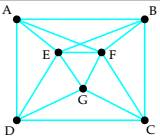# (a) determine whether the graph is Eulerian. If it is, find an Euler circuit. If it is not, explain why. (b)If the graph does not have an Euler circuit, does it have an Euler path? If so, find one. If not, explain why.### Mathematical Excursions (MindTap C...

4th Edition
Richard N. Aufmann + 3 others
Publisher: Cengage Learning
ISBN: 9781305965584

#### Solutions

Chapter
Section### Mathematical Excursions (MindTap C...

4th Edition
Richard N. Aufmann + 3 others
Publisher: Cengage Learning
ISBN: 9781305965584
Chapter 5.1, Problem 21ES
Textbook Problem
1 views

## (a) determine whether the graph is Eulerian. If it is, find an Euler circuit. If it is not, explain why. (b)If the graph does not have an Euler circuit, does it have an Euler path? If so, find one. If not, explain why.To determine

To determine if the graph is Eulerian. If it is, to find an Euler circuit else to explain why not. And if the graph does not have an Euler circuit, to check if it has an Euler path. If it has to find it else to explain the reason for not having an Euler path.

### Explanation of Solution

Given information:

Given graph is

Calculation:

A graph is said to be Eulerian graph if it is a connected graph and every vertex of the graph has even degree.

Here,

The degree of A is 4

The degree of B is 4

The degree of C is 4

The degree of D is 4

The degree of E is 5

The degree of F is 5

The degree of G is 4

Since the graph is connected and all the vertices do not have even degree, the graph is not Eulerian.

A circuit (that starts and ends at the same vertex) is said to be Euler circuit if it uses every edge, but only once. (i.e. no edge is used more than once)...

### Still sussing out bartleby?

Check out a sample textbook solution.

See a sample solution

#### The Solution to Your Study Problems

Bartleby provides explanations to thousands of textbook problems written by our experts, many with advanced degrees!

Get Started

Find more solutions based on key concepts
In Exercises 1-8, use the graph of the given function f to determine limxaf(x) at the indicated value of a, if ...

Applied Calculus for the Managerial, Life, and Social Sciences: A Brief Approach

Identify the four steps of a hypothesis test as presented in this chapter.

Statistics for The Behavioral Sciences (MindTap Course List)

If A=1,2,3,4 and B=2,4,6,8, find: a NA b NB c NAB d NAB

Elementary Geometry For College Students, 7e

In Exercises 57-62, Use Venn diagram to illustrate each statement. A(BC)=(AB)C

Finite Mathematics for the Managerial, Life, and Social Sciences

For , which vector, a, b, c, or d, best represents F(1, 1)? a b c d

Study Guide for Stewart's Multivariable Calculus, 8th

Reminder Round all answers to two decimal places unless otherwise indicated. Cost of TransportPhysiologists hav...

Functions and Change: A Modeling Approach to College Algebra (MindTap Course List)

Explain the difference among the terms research strategy, research design, and research procedure.

Research Methods for the Behavioral Sciences (MindTap Course List)

The maximum area in question 2 is ft2 20 ft2 25ft2 50 ft2 A carpenter has a 10-foot-long board to mark off a t...

Study Guide for Stewart's Single Variable Calculus: Early Transcendentals, 8th